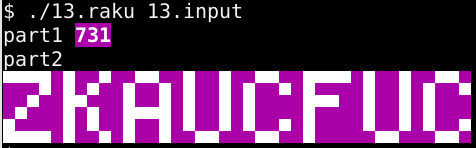TL;DR

On with Advent of Code puzzle 13 from 2021: folding transparent paper, origami style.

This day’s puzzle(s) were approachable for my Raku level, which was good because I got to practice a bit without having to resort too much to the mighty internet.

The starting part is the usual one:

#!/usr/bin/env raku
use v6;

sub MAIN ($filename =$?FILE.subst(/\.raku$/, '.tmp')) { my$inputs = get-inputs($filename); my ($part1, $part2) = solve($inputs);

my $highlight = "\e[1;97;45m"; my$reset     = "\e[0m";
put "part1 $highlight$part1$reset"; put "part2\n$highlight$part2$reset";
}

sub solve ($inputs) { return (part1($inputs), part2($inputs)); }  Parsing the inputs is done sub-optimally because it has two parts with two different formats. I could have split into two paragraphs and then parse each of them separately, but decided to go for a state machine. sub get-inputs ($filename) {
my @board;
my @folds;
my $do-board = 1; for$filename.IO.lines -> $line { if$line ~~ /^ \s* $/ {$do-board = 0;
}
elsif $do-board { @board.push: [$line.comb: /\d+/];
}
else {
my @fold = 0, 0;
$line ~~ / (.) \= (\d+) /; if$0 eq 'x' { @fold = +$1 } else { @fold = +$1 }
@folds.push: @fold;
}
}
return {board => @board, folds => @folds};
}


Both parts of the solution rely on a folding operation, so let’s bite the bullet right now:

sub fold ($board,$fold) {
$board.map(->$p {
(0 .. $fold.end).map({$p[$_] <=$fold[$_] ??$p[$_] !! 2 *$fold[$_] -$p[$_]; }).join(',') }).Set.keys».comb(/\d+/)».Array; }  The function above applies one single $fold operation onto $board. Each direction is folded independently, allowing (theoretically) for folds over two axes at the same time. Not our case anyway. The folding operation itself goes like this: • if the value$v$is within the remaining part, it is kept; • otherwise it is mirrored with respect to the fold position$f$. This would mean getting the value$f - (v - f) = 2f - v$. The resulting points are stringified (with join), then passed through a Set and keys to remove duplicates. This leaves us the unique remaining points, still represented as strings. For this reason, each string is split back into numeric values via comb and encapsulated inside (small) arrays. The first part is about applying the first fold and counting how many (unique) points we are left with. This is now trivial because our fold function already removed duplicates for us: sub part1 ($inputs) {
my @folded = fold($inputs<board>,$inputs<folds>);
return @folded.elems;
}


The second part is just going through all folds in sequence, then render the output. We will not be doing any fancy automatic recognition of the letters, but we will at least print them with (some) style:

sub part2 ($inputs) { my$board = $inputs<board>;$board = fold($board,$_) for $inputs<folds>.List; my ($mx, $my) X= 0; for @$board -> $p {$mx = max($mx, +$p);
$my = max($my, +$p); } my @rendered = (0 .. ($my / 2).Int).map({[' ' xx (1 + $mx)]}); for @$board -> $p { my$y = ($p / 2).Int; if @rendered[$y][$p] ne ' ' { @rendered[$y][$p] = "\c[FULL BLOCK]"; } elsif$p %% 2 {
@rendered[$y][$p] = "\c[UPPER HALF BLOCK]";
}
else {
@rendered[$y][$p] = "\c[LOWER HALF BLOCK]";
}
}
return @rendered».join('').join("\n");
}


This is what I mean by style:Well… enough for this puzzle, see you and stay safe!

Comments? Octodon, , GitHub, Reddit, or drop me a line!